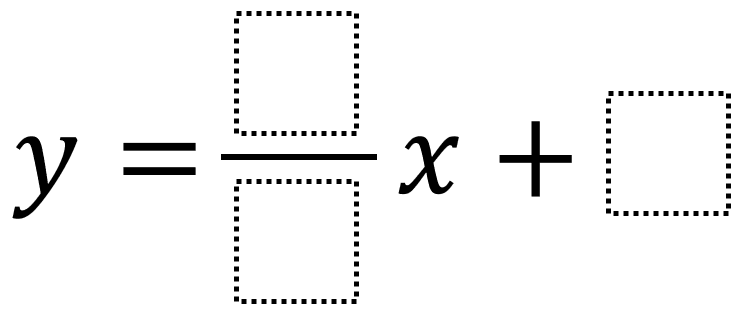# Graphing Linear Equations 2

Directions: Using the integers -9 to 9 at most one time each, fill in the boxes to make a linear equation which goes through (5, 4) and has a slope that’s as close to 0 as possible without being horizontal.### Hint

How can you determine whether your line intersects the point (5, 4) algebraically? How can you determine whether your line intersects the point (5, 4) graphically? Here’s a link to an already set up Desmos version. How can you determine if your linear equation’s slope is closer to 0 than another student’s slope?

The slope of the line that goes through (5, 4) and has a slope that is as close to 0 as possible without being horizontal is y = (-2/5)x + 6.

Source: Robert Kaplinsky

## Exponential Powers

Directions: Using the digits 0 to 9 at most one time each, place a digit …

### One comment

1.The answer is not the above answer. It is y = (1/5)x + 3